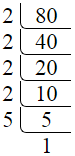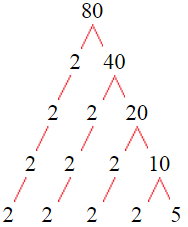# Factors of 80The factors of 80 are 1, 2, 4, 5, 8, 10, 16, 20, 40, and 80 i.e. F80 = {1, 2, 4, 5, 8, 10, 16, 20, 40, 80}. The factors of 80 are all the numbers that can divide 80 without leaving a remainder.

We can check if these numbers are factors of 80 by dividing 80 by each of them. If the result is a whole number, then the number is a factor of 80. Let's do this for each of the numbers listed above:

·        1 is a factor of 80 because 80 divided by 1 is 80.

·        2 is a factor of 80 because 80 divided by 2 is 40.

·        4 is a factor of 80 because 80 divided by 4 is 20.

·        5 is a factor of 80 because 80 divided by 5 is 16.

·        8 is a factor of 80 because 80 divided by 8 is 10.

·        10 is a factor of 80 because 80 divided by 10 is 8.

·        16 is a factor of 80 because 80 divided by 16 is 5.

·        20 is a factor of 80 because 80 divided by 20 is 4.

·        40 is a factor of 80 because 80 divided by 40 is 2.

·        80 is a factor of 80 because 80 divided by 80 is 1.

## How to Find Factors of 80?

1 and the number itself are the factors of every number. So, 1 and 80 are two factors of 80. To find the other factors of 80, we can start by dividing 80 by the numbers between 1 and 80. If we divide 80 by 2, we get a remainder of 0. Therefore, 2 is a factor of 80. If we divide 80 by 3, we get a remainder of 2. Therefore, 3 is not a factor of 80.

Next, we can check if 4 is a factor of 80. If we divide 80 by 4, we get a remainder of 0. Therefore, 4 is also a factor of 80. We can continue this process for all the possible factors of 80.

Through this process, we can find that the factors of 80 are 1, 2, 4, 5, 8, 10, 16, 20, 40, and 80. These are the only numbers that can divide 80 without leaving a remainder.

********************

********************

## Properties of the Factors of 80

The factors of 80 have some interesting properties. One of the properties is that the sum of the factors of 80 is equal to 186. We can see this by adding all the factors of 80 together:

1 + 2 + 4 + 5 + 8 + 10 + 16 + 20 + 40 + 80 = 186

Another property of the factors of 80 is that the prime factors of 80 are 2, and 5 only.

## Applications of the Factors of 80

The factors of 80 have several applications in mathematics. One of the applications is in finding the highest common factor (HCF) of two or more numbers. The HCF is the largest factor that two or more numbers have in common. For example, to find the HCF of 80 and 24, we need to find the factors of both numbers and identify the largest factor they have in common. The factors of 80 are 1, 2, 4, 5, 8, 10, 16, 20, 40, and 80. The factors of 24 are 1, 2, 3, 4, 6, 8, 12, and 24. The largest factor that they have in common is 8. Therefore, the HCF of 80 and 24 is 8.

Another application of the factors of 80 is in prime factorization. Prime factorization is the process of expressing a number as the product of its prime factors. The prime factors of 80 are 2, and 5 since these are the only prime numbers that can divide 80 without leaving a remainder. Therefore, we can express 80 as:

80 = 2 × 2 × 2 × 2 × 5

We can do prime factorization by division and factor tree method also. Here is the prime factorization of 80 by division method,80 = 2 × 2 × 2 × 2 × 5

Here is the prime factorization of 80 by the factor tree method,80 = 2 × 2 × 2 × 2 × 5

## Conclusion

The factors of 80 are the numbers that can divide 80 without leaving a remainder. The factors of 80 are 1, 2, 4, 5, 8, 10, 16, 20, 40, and 80. The factors of 80 have some interesting properties, such as having a sum of 186. The factors of 80 have several applications in mathematics, such as finding the highest common factor and prime factorization.